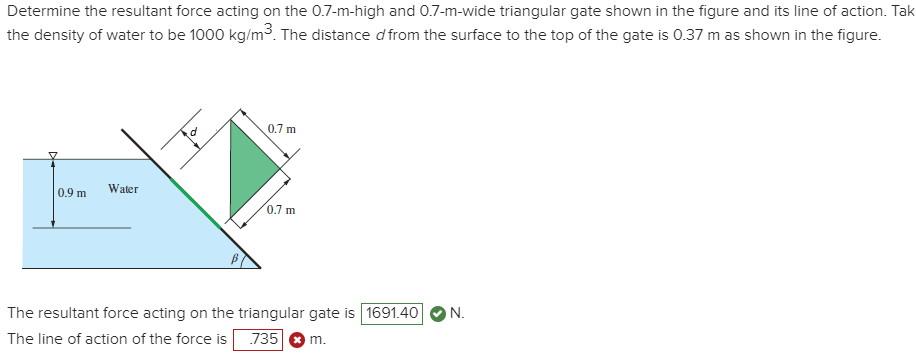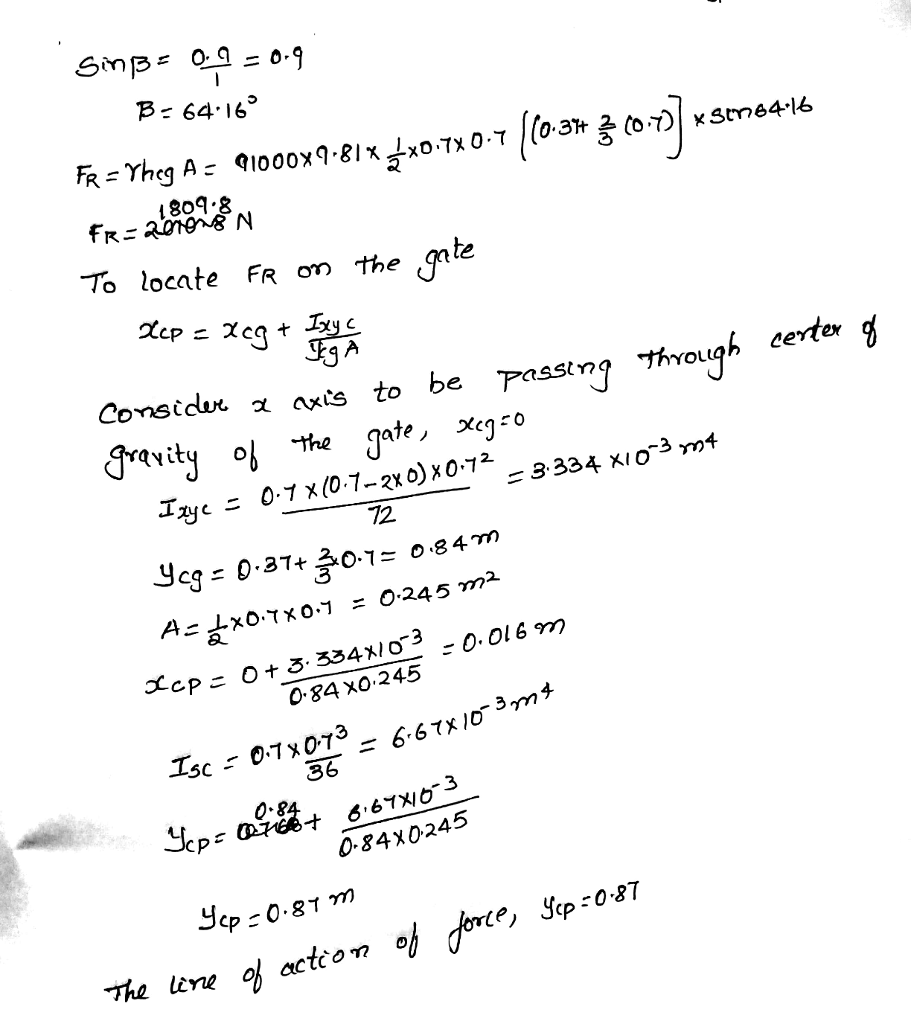# Determine the resultant force acting on the 0.7-m-high and 0.7-m-wide triangular gate shown in the figure and its line of action. Tak the density of water to be 1000 kg/m3. The distance d from the surface to the top of the gate is 0.37 m as shown in the figure.

Question-AnswerCategory: Fluid MechanicsDetermine the resultant force acting on the 0.7-m-high and 0.7-m-wide triangular gate shown in the figure and its line of action. Tak the density of water to be 1000 kg/m3. The distance d from the surface to the top of the gate is 0.37 m as shown in the figure.

Determine the resultant force acting on the 0.7-m-high and 0.7-m-wide triangular gate shown in the figure and its line of action. Tak the density of water to be 1000 kg/m3. The distance d from the surface to the top of the gate is 0.37 m as shown in the figure.